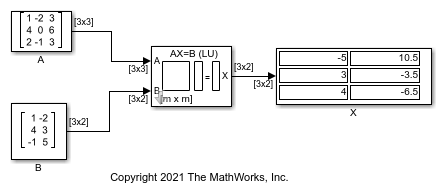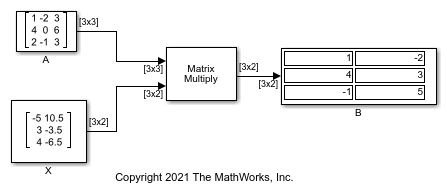# Solve Matrix Equation Using LU Solver

Solve a matrix equation using the LU Solver block.

Open and run the model. The model solves the equation AX = B using the LU Solver block. The block uses the A and B matrices as inputs and outputs the solution matrix X.You can verify the solution by using the Matrix Multiply block to perform the multiplication AX, as shown in the following model. The output matrix B in this model equals the input matrix B in the previous model.